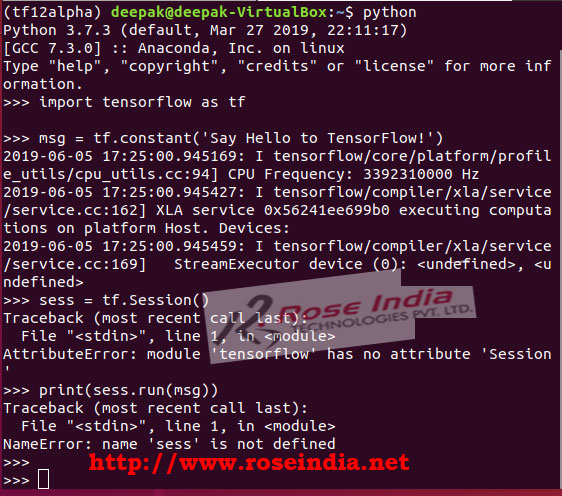# TensorFlow 2.0 Hello World

In this section of TensorFlow tutorials series we will first develop a TensorFlow 2.0 Hello World program. This is the first program in TensorFlow which will give you idea about running a program in TensorFlow.

In this section of TensorFlow tutorials series we will first develop a TensorFlow 2.0 Hello World program. This is the first program in TensorFlow which will give you idea about running a program in TensorFlow.

## TensorFlow 2.0 Hello World - Writing your first TensorFlow 2.0 example

In this tutorial we will learn to write TensorFlow 2.0 Hello World which is the first program you should learn develop in TensorFlow 2.0. There are lot of changes in the TensorFlow 2.0 as compared to the previous TensorFlow 1.x releases. TensorFlow comes with the ease of development less coding it need in this version of TensorFlow. The TensorFlow 2.0 is developed to remove the issues and complexity of previous versions.

The removal of ts.Session() is one of the important thing and if you are moving your code from TensorFlow 1.x then new methods needs to be used. In the TensorFlow 2.0 eager execution is enabled by default. The eager execution mode evaluates the program immediately and without building the graph. The eager execution mode operation returns the concrete value instead of constructing a computational graph.

Here is sample code of TensorFlow 1.x Hello World application:

``````
import tensorflow as tf
msg = tf.constant('Say Hello to TensorFlow!')
sess = tf.Session()
print(sess.run(msg))
```
```

Here we have to use the tf.Session() to run the computational graph. Now the tf.Session() is removed from TensorFlow 2.0 and we can't use it. If you run this program in TensorFlow 2.0 it will throw following error:

```>>> sess = tf.Session()
Traceback (most recent call last):
File "<stdin>", line 1, in <module>
AttributeError: module 'tensorflow' has no attribute 'Session'
>>> print(sess.run(msg))
Traceback (most recent call last):
File "<stdin>", line 1, in <module>
NameError: name 'sess' is not defined

```

Here is screen shot:To write the program in TensorFlow 2.0 we have to remove tf.Session() and then use tf.print() to print the value of constant. Since, TensorFlow 2.0 is executing in eager mode no computational graph is created and TensorFlow 2.0 executes the code line by line.

Here is example of TensorFlow 2.0 Hello World program:

``````
import tensorflow as tf
msg = tf.constant('TensorFlow 2.0 Hello World')
tf.print(msg)
```
```

Here is the output of program execution:

```(tf12alpha) [email protected]:~\$ python
Python 3.7.3 (default, Mar 27 2019, 22:11:17)
[GCC 7.3.0] :: Anaconda, Inc. on linux
>>> import tensorflow as tf

>>> msg = tf.constant('TensorFlow 2.0 Hello World')
2019-06-05 18:13:09.850002: I tensorflow/core/platform/profile_utils/cpu_utils.cc:94] CPU Frequency: 3392310000 Hz
2019-06-05 18:13:09.850258: I tensorflow/compiler/xla/service/service.cc:162] XLA service 0x557cc1455bc0 executing computations on platform Host. Devices:
2019-06-05 18:13:09.850296: I tensorflow/compiler/xla/service/service.cc:169]   StreamExecutor device (0):
>>> tf.print(msg)
TensorFlow 2.0 Hello World
```

Screen shot of TensorFlow 2.0 Hello World program execution:Above example shows the execution of TensorFlow 2.0 Hello World program.

In TensorFlow 2.0 session has been removed and the eager execution is true by default. TensorFlow 2.0 simplified coding of machine learning programs.

Check more tutorials at: